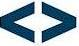## Lambda Expression in Java SE 8

Introduction

In Object Oriented programming languages, object is the first class citizen but reverse is the case in functional programming languages because functions are first class citizens in which they can be passed around as if they are variables this is seen in Haskell, javascript and other functional languges. Functional languages have other constructs that are not readily available in object oriented languages such as closure, lambda expressions and so on.

Lambda expression was not part of earlier versions of Java and as such, passing method or function as arguments was normally achieved through the use of anonymous class which was not elegant. But with the introduction of Lambda Expression in Java 8 the language can be made to behave like a functional language, though not with closure anywa. Thus, the introduction of Lambda Expression in Java 8 in a way bridges the gap the language has with functional programming language.

Lambda Expression is usually referred to as anonymous function, a function without a name. It is a shorthand that lets you declare a method where it is going to be used without specifying the method name, return type, access specifier. It enables you to treat code as data and use function as method arguments.

Why you should care about Lambda Expression
1. The introduction of lambda expression in java will make available the benefits of functional language in java.
2. Lambda expression provides a means to write clear and concise codes with the use of expressions.
3. Collection filtering, Iteration and Extraction can be greatly improved using Lambda expression.

NB: JDK 8 or a higher version is required

Syntax

```
(argument) -> (body)
```

Where there can be zero or more arguments, The arrow token is the java lambda operator and (body) contains one or more line of codes to be executed when the function is called.

Examples

```(int a, int b) - > a*b
(Person p) - > { return p.getAge();}
```
In the first example, the expression's arguments are the integer values a and b which would be used by the expression to return the output of the multiplication operation.

The second example takes an object person as argument while the expression returns the value from the getAge() method called on the object.

Prior to Java 8, codes to handle EventListener in swing were normally written using the anonymous class
```btnOk.setOnAction( newEventHandler<ActionEvent>() {
@Override
public voidhandle(ActionEvent e) {
// codes to handle button event would be written here
}
});
```
With lambda expression, the EventListener code can be made to be elegant with less lines of codes as shown below
```btnOk.setOnAction( e -> {
// codes to handle button event would be written here
});

```
Another example can be seen in Thread code where anonymous class codes can now be substituted for that of a lambda expression
```
() -> System.out.println("This is a seperate thread")
).start();
```
Furthermore, Lambda Expression can be used in collections where it can be used to simplify some operations such as filtering, sorting and even querying of items in a collection.
```    ArrayList<Integer> arrayList= new ArrayList<>();
for(int i=1; i<=100; i++)

//without lambda expression
for(Integer i: arrayList){
System.out.println( i);
}

//with lambda expression
arrayList.forEach( i -> {System.out.println(i);});
```
From the codes above, it is glaring that lambda expression minimizes the lines of codes to be written, thus making it elegant.

In this blog post, I have been able to show how Lambda Expression can be made to bridge the gap between java and functional languages, while reducing the lines of code written in the process.

0 People Like(s) This PagePermalink